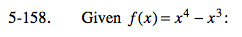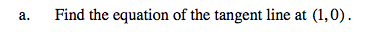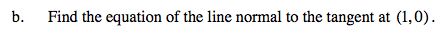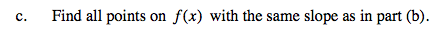### Home > CALC > Chapter 5 > Lesson 5.5.1 > Problem5-158

5-158.Use the point-slope formula.

Use f '(x) to find the slope at x = 1. You already know the point.Normal lines are means perpendicular to the tangent line at the point of tangency.Let f(x) = the slope in part (b). Solve for x. Then find the corresponding y-value(s). Write your answer(s) as coordinate point(s).